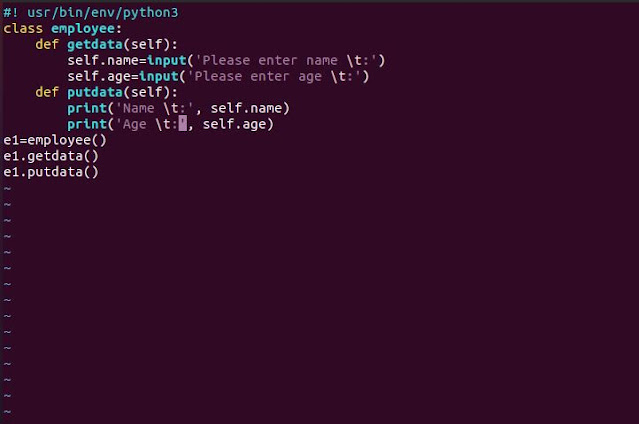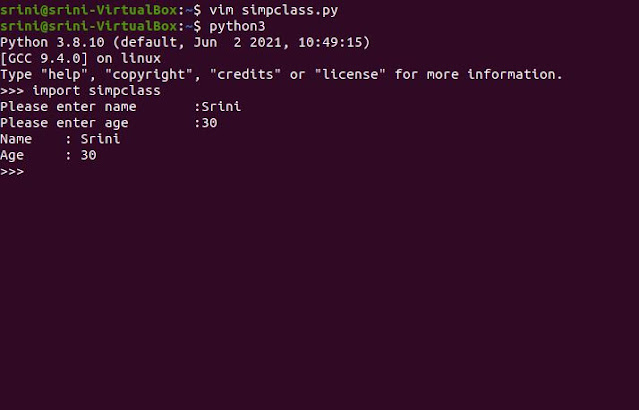# The Real Reason Self Argument in Python

Here is the top reason to pass the 'self' argument in the Python class function. You already know that the python class has two things. One is function and the other one is members.## Structure of a class

class <name of the class>:
def <function name>(<arguments>):
...
<members>

For example, the employee class having data members' names and ages and member functions getdata() and putdata() can be defined as below.

Every function in the class must have at least one argument, self. The data members are accessed via the self-object as they belong to the class and not just the function. Likewise, the putdata() function displays the values of the data members.

Notes on Python class
• A class definition has functions but can also have other members.
• The attribute of an object is a data attribute, and the function that belongs to an object is the method.

## Python class: sample program

class employee:
def getdata(self):
self.name=input('Enter name\t:')
self.age=input('Enter age\t:')
def putdata(self):
print('Name\t:',self.name)
print('Age\t:',self.age)
e1=employee()
e1.getdata()
e1.putdata()## Testing: The output from the class program

Here are the commands that I used to test the python class quickly. Just I Imported my .py  module to the Python3 shell. It ran automatically. One main point, while importing, if syntax errors are there, those you need to fix.References